×#### Thank you for registering.

One of our academic counsellors will contact you within 1 working day.

Click to Chat

1800-1023-196

+91-120-4616500

CART 0

• 0

MY CART (5)

Use Coupon: CART20 and get 20% off on all online Study Material

ITEM
DETAILS
MRP
DISCOUNT
FINAL PRICE
Total Price: Rs.

There are no items in this cart.
Continue Shopping• Complete Physics Course - Class 11
• OFFERED PRICE: Rs. 2,968
• View Details

```Cells in Series and in Parallel

Table of Content

The beginning of cells

Cell

Series and Parallel Connections

Combination of Cells in Series Connection

Combination of Cells in Parallel Connection

Summary

The beginning of cells

Alessandro Volta invented electric battery. It was first named as Voltaic Pile. For his contributions to science, the unit of electric potential is named as Volt. John Frederic Daniell developed Daniell cell. Then George Leclanche invented Wet cell and Dr. Carl Gassner introduced the Dry cell battery. Gaston Plante introduced the first rechargeable battery. It is the lead-acid battery and is again most common application found in cars.

Cell

We know that electrical current is the flow of charged particles. It is the flow of electrons through a circuit.

A collection of two or more cells which are connected in series is called A Battery. A battery is an energy source that converts chemical energy to electrical energy. It is otherwise known as Electrochemical Cell. The energy is stored in the form of chemical energy inside a battery. Batteries give us a convenient source of energy for energizing devices without cables and wires. When it is connected to a circuit it produces electrical energy. A battery consists of two terminals – A Positive and Negative Terminal. The positive terminal is called Cathode and the negative terminal is called Anode. They are also called as Electrodes of a Cell. These electrodes will be dipped in a solution called Electrolyte. It is liquid which is ionic and conducts electricity.

When the battery is about to charge, an external source is connected to it. The anode of the battery is connected to the negative terminal of the source and cathode is connected to the positive terminal of the source. As the external source is connected to the battery, electrons are inserted into the anode. When the cell or battery is connected to the circuit chemical reactions takes place. Thus chemical reactions takes place within the two electrodes. Here oxidation and reduction reactions happens. Then reduction reaction occurs at cathode and oxidation process occurs at anode.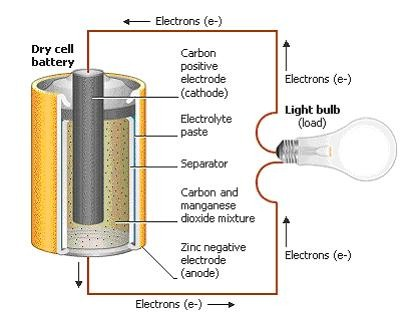A dry cell

The cathode act as the oxidizing agent by accepting electrons from the negative terminal anode. The anode act as the reducing agent by losing the electrons. Thus due to these chemical reactions an electrical difference occurs between the terminals-anode and cathode. When there is no power the electrolyte prohibits the movement of electrons directly from anode to cathode. This is why we are using an external source or connecting to a circuit. Thus electrons travel from anode to cathode when the circuit is closed. Finally it gives power to the appliance which is connected to it. After a long time when the electrochemical process alters the anode and cathode materials it stop giving out electrons. Then the battery dies.

EMF:

EMF or Electromotive force is defined as the potential difference which is developed between the two terminals of a battery in an open circuit. We know that anode has positive potential (V+) and cathode has negative potential (V-). So emf is the potential difference between the positive terminal anode and negative terminal cathode when there is no current flowing through it. The emf measures the energy which is transferred to the charge carries in the cell or a battery. It is the energy in joules divided by the charge in coulombs. The emf acts as the initiating force for the current to flow.

ε = E/Q, where ε is the electromotive force, E is the energy and Q is the charge.

The emf which is denoted by ε and the equation is given by ε = V+ - (-V-) = V+ + V-. It is measured in volts.

Internal Resistance:

Internal Resistance is the resistance which is present within the battery that resists the current flow when connected to a circuit. Thus, it causes a voltage drop when current flows through it. It is the resistance provided by the electrolyte and electrodes which is present in a cell. So Internal resistance is offered by the electrodes and electrolyte which oppose the current flow inside the cell.

Emf and Internal Resistance Equation:

Consider the circuit given below. The cell can be modified with an emf ε and the internal resistor with resistance r which is connected in series. An external load resistor with resistance R is also connected across the circuit. The terminal potential difference represented as V is defined as the potential difference developed between the positive and negative terminals of the cell when current flows through the circuit.

V = V+ + V- – Ir. This is the voltage drop accomplished due to the internal resistance.

We know that ε = V+ + V-. = I (R + r).

ε = IR + Ir.

= V + Ir

V = ε – Ir.

So, V = ε – Ir, where V is the potential difference across the circuit, ε is the emf, I is the current flowing through the circuit, r is internal resistance.

Usually internal resistance of a cell is not considered because ε >> Ir. The value of internal resistance changes from cell to cell.

Series and Parallel Connections

There are mainly two types of circuits, series and parallel. Cells can be connected both in series, parallel or a combination of both. In series circuit electrons travel only in one path. Here the current will be the same which passes through each resistor. The voltage across resistors in a series connection will be different. Series circuits do not overheat easily. The design of series circuit is simple compared to parallel circuits.

In parallel circuit electrons travel through many branches in it. In this case, the voltage remains the same across each resistor in the circuit. Here the current in the circuit is divided among each branch and finally recombines when the branches meet at a common point. A parallel circuit can be formed in many ways, which means cells can be arranged in different forms. Parallel circuits can be used as a current divider. It is easy to connect or disconnect a new cell or other component without affecting the other elements in the parallel circuit. But it uses a lot of wires and hence becomes complex.

Combination of Cells in Series Connection

Consider two cells which is connected in series. The positive terminal of one cell is connected to negative terminal of the next cell. Here one terminal of two cells are free and the other terminal of two cells are joined together. ε1 and ε2  are the emfs of the cells and r1 and r2 are the internal resistance of the cells respectively. Let I be the current flowing through the cells.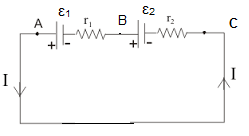Cells connected in series

Consider the points A, B and C and let V (A), V (B) and V (C) be the potentials of these points respectively.  V (A) - V (B) will be the potential difference between the positive and negative terminals for the first cell.

So VAB = V (A) - V (B) = ε1- Ir1.

VBC = V (B) - V (C) = ε2 – Ir2.

Now the potential difference between the terminals A and C is

VAC  = V (A) – V(C) = [V (A) - V (B)] + V (B) - V (C)]

= ε1- Ir1 + ε2 – Ir2

= ( ε1 + ε2) – I(r1+r2).

In case if we replace this combination of cells by a single cell between the points A and C with emf εeq and internal resistance req, VAC = εeq - req. and thus we found out that  εeq = ε1 + ε2  and req = r1+r2 from the previous equation.

It is clear that the equivalent emf of n number of cells in series combination is the sum of their individual emfs. The equivalent internal resistance of n cells in series combination is the sum of their individual internal resistance.

In series combination if the current leaves the cell from the negative electrode, the emf of the cell will be for example VBC = - ε2 – Ir2 and finally the equation for εeq = ε1 - ε2, (ε1 > ε2).

The cells connected in series produces a greater resultant voltage. The cells which are damaged can be easily identified and hence can be replaced easily as they break the circuit.

If any one of the cell is damaged in the circuit, it may affect the whole connection. The cells which are connected in series gets easily exhausted and so they do not last longer. It is not used in house wiring.

Combination of Cells in Parallel Connection

Consider two cells which is connected in parallel. Here the positive terminals of all cells are connected together and negative terminals of all cells are connected together. In parallel connection, the current is divided among the braches. Thus, the current I is split into I1 and I2. I = I1 + I2.  Consider the points B1 and B2 and then V (B1) and V (B2) are the potentials respectively. The potential difference across the terminals of the first cell.Cells connected in parallel

V = V (B1) - V (B2) = ε1- I1 r1. The point B1 and B2  are connected similar to the second cell.

V = V (B1) - V (B2) = ε2 – I2 r2. By ohm’s law we know that I = V / R. Now substitute these values in the equation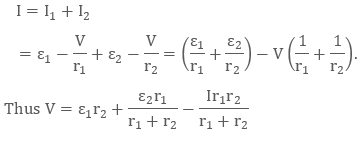If we replace the cells by a single cell lying between the point B1 and B2 with emf εeq  and internal resistance req, then V = εeq - Ireq.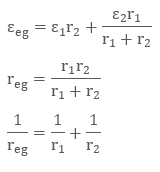It is the same as when we connect the resistors in parallel connection.For n number of cells connected in parallel with emf ε1, ε2…… εn  and internal resistance r1, r2…. rn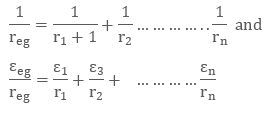For the cells connected in parallel if any one of the cell is damaged in the circuit, it will not affect the whole connection. The cells which are connected in parallel do not exhaust easily and thus they last longer.

The voltage developed by the cells in parallel connection cannot be increased by increasing the number of cells present in the circuit. It is because they do not have same circular path. In parallel connection the connection provides power based on one cell. So the brightness of the bulb will not be high.

Summary

It was Alessandro Volta who invented electric battery and was first named as voltaic pile.

Frederic Daniell developed Daniell cell and George Leclanche invented Wet cell. Dr. Carl Gassner introduced the Dry cell battery and Gaston Plante introduced the first rechargeable battery.

A cell or a battery is an energy source that converts chemical energy to electrical energy.

A battery consists of two terminals. The positive terminal is called Cathode and the negative terminal. is called Anode.

EMF or electromotive force is the potential difference developed between the two terminals of a battery in an open circuit. ε = E/Q, where ε is the electromotive force, E is the energy and Q is the charge. Internal resistance is the resistance which is present within the battery that resists the current flow when connected to a circuit. The equation connecting the emf and internal resistance isV = ε – Ir, where V is the potential difference across the circuit, ε is the emf, I is the current flowing through the circuit, r is the internal resistance

For the two cells connected in series the voltage developed is V = (ε1 + ε2) – I (r1+r2). V = εeq - req if we replace the number of cells by a single cell.

For the two cells connected in parallel V = ε1 r2 + ε2 r1/ r1+ r2 – Ir1r2 / r1+ r2. For n number of cells connected in parallel εeq / req = ε1 / r1 + ε2 / r2 +…………………….. εn / rn.

Watch this Video for more reference

Cells in Series and in Parallel
```### Course Features

• 101 Video Lectures
• Revision Notes
• Previous Year Papers
• Mind Map
• Study Planner
• NCERT Solutions
• Discussion Forum
• Test paper with Video Solution